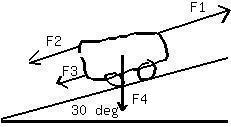# A 292 kg motorcycle is accelerating up along a ramp that is inclined 30.0° above the horizontal. The propulsion force pushing the motorcycle up the raFalak Kinney 2020-11-05 Answered
A 292 kg motorcycle is accelerating up along a ramp that is inclined 30.0° above the horizontal. The propulsion force pushing the motorcycle up the rampis 3020 N, and air resistance produces a force of 250 N that opposes the motion. Find the magnitude of the motorcycles
You can still ask an expert for help

• Questions are typically answered in as fast as 30 minutes

Solve your problem for the price of one coffee

• Math expert for every subject
• Pay only if we can solve itLaaibah Pitt

What are the sum of forces on the motorcycle? Theproblem states the following:
The motorcycle is pushed up the ramp with force 3020 N
Air resists the motorcyles forward motion, therefore it pusheswith a force of 250 N down the ramp.
The only other force is that due to the motorcycles weight(a. force of gravity). Then the force diagram looks like);Where;
F1=3020 Newtons
F3=250 Newtons
F4 is force due to gravity and F2 is the component of that force parallel to the ramp.
So,
${F}_{2}=\left(292\right)\left(9.8\right)\mathrm{sin}\left(30\right)=1430.8$
now the sum of the forces is equal to the mass times acceleration of the motorcycle.
$\sum F=F1-F2-F3$ or
Then the acceleration of the motorcycle is:
up the slope
The second part of the problem is also done by summing forces. The force of the block on the surface is due to the mass of the block and mass of the person standing on it. Thisforce must be exactly opposed by the surface (since the block is not moving in the vertical direction).
Then the normal force is:
${F}_{n}=\left({m}_{1}+{m}_{2}\right)g;$ where g is the acceleration to gravity.
${F}_{n}=\left(41+69\right)\cdot 9.8=1078$ Newtons, perpendicular to thesurface and pointing up
Similarly, the person applies a force to the block surface (due to weight) and the block surface response with equal and opposite force:
${F}_{n}=69\cdot 9.8=676.2$ Newtons, perpendicular to block surfaceand pointing up.